oomph::FaceGeometry< TPoissonElement< 1, NNODE_1D > > Class Template Reference

Face geometry for the 1D TPoissonElement elements: Point elements. More...

#include <Tpoisson_elements.h>Inheritance diagram for oomph::FaceGeometry< TPoissonElement< 1, NNODE_1D > >:

## Public Member Functions

FaceGeometry ()
Constructor: Call the constructor for the appropriate lower-dimensional TElement. More...Public Member Functions inherited from oomph::PointElement
PointElement ()
Constructor. More...

PointElement (const PointElement &)=delete
Broken copy constructor. More...

void shape (const Vector< double > &s, Shape &psi) const
Broken assignment operator. More...

void local_coordinate_of_node (const unsigned &j, Vector< double > &s) const
Get local coordinates of node j in the element; vector sets its own size. More...Public Member Functions inherited from oomph::FiniteElement
void set_dimension (const unsigned &dim)
Set the dimension of the element and initially set the dimension of the nodes to be the same as the dimension of the element. More...

void set_nodal_dimension (const unsigned &nodal_dim)
Set the dimension of the nodes in the element. This will typically only be required when constructing FaceElements or in beam and shell type elements where a lower dimensional surface is embedded in a higher dimensional space. More...

void set_nnodal_position_type (const unsigned &nposition_type)
Set the number of types required to interpolate the coordinate. More...

void set_n_node (const unsigned &n)
Set the number of nodes in the element to n, by resizing the storage for pointers to the Node objects. More...

int nodal_local_eqn (const unsigned &n, const unsigned &i) const
Return the local equation number corresponding to the i-th value at the n-th local node. More...

double dJ_eulerian_at_knot (const unsigned &ipt, Shape &psi, DenseMatrix< double > &djacobian_dX) const
Compute the geometric shape functions (psi) at integration point ipt. Return the determinant of the jacobian of the mapping (detJ). Additionally calculate the derivatives of "detJ" w.r.t. the nodal coordinates. More...

FiniteElement ()
Constructor. More...

virtual ~FiniteElement ()
The destructor cleans up the static memory allocated for shape function storage. Internal and external data get wiped by the GeneralisedElement destructor; nodes get killed in mesh destructor. More...

FiniteElement (const FiniteElement &)=delete
Broken copy constructor. More...

virtual bool local_coord_is_valid (const Vector< double > &s)
Broken assignment operator. More...

virtual void move_local_coord_back_into_element (Vector< double > &s) const
Adjust local coordinates so that they're located inside the element. More...

void get_centre_of_gravity_and_max_radius_in_terms_of_zeta (Vector< double > &cog, double &max_radius) const
Compute centre of gravity of all nodes and radius of node that is furthest from it. Used to assess approximately if a point is likely to be contained with an element in locate_zeta-like operations. More...

virtual void local_fraction_of_node (const unsigned &j, Vector< double > &s_fraction)
Get the local fraction of the node j in the element A dumb, but correct default implementation is provided. More...

virtual double local_one_d_fraction_of_node (const unsigned &n1d, const unsigned &i)
Get the local fraction of any node in the n-th position in a one dimensional expansion along the i-th local coordinate. More...

virtual void set_macro_elem_pt (MacroElement *macro_elem_pt)
Set pointer to macro element – can be overloaded in derived elements to perform additional tasks. More...

MacroElementmacro_elem_pt ()
Access function to pointer to macro element. More...

void get_x (const Vector< double > &s, Vector< double > &x) const
Global coordinates as function of local coordinates. Either via FE representation or via macro-element (if Macro_elem_pt!=0) More...

void get_x (const unsigned &t, const Vector< double > &s, Vector< double > &x)
Global coordinates as function of local coordinates at previous time "level" t (t=0: present; t>0: previous). Either via FE representation of QElement or via macro-element (if Macro_elem_pt!=0). More...

virtual void get_x_from_macro_element (const Vector< double > &s, Vector< double > &x) const
Global coordinates as function of local coordinates using macro element representation. (Broken virtual — this must be overloaded in specific geometric element classes) More...

virtual void get_x_from_macro_element (const unsigned &t, const Vector< double > &s, Vector< double > &x)
Global coordinates as function of local coordinates at previous time "level" t (t=0: present; t>0: previous). using macro element representation (Broken virtual – overload in specific geometric element class if you want to use this functionality.) More...

virtual void set_integration_scheme (Integral *const &integral_pt)
Set the spatial integration scheme. More...

Integral *const & integral_pt () const
Return the pointer to the integration scheme (const version) More...

virtual void shape_at_knot (const unsigned &ipt, Shape &psi) const
Return the geometric shape function at the ipt-th integration point. More...

virtual void dshape_local (const Vector< double > &s, Shape &psi, DShape &dpsids) const
Function to compute the geometric shape functions and derivatives w.r.t. local coordinates at local coordinate s. This function must be overloaded for each specific geometric element. (Broken virtual function — specifies the interface) More...

virtual void dshape_local_at_knot (const unsigned &ipt, Shape &psi, DShape &dpsids) const
Return the geometric shape function and its derivative w.r.t. the local coordinates at the ipt-th integration point. More...

virtual void d2shape_local (const Vector< double > &s, Shape &psi, DShape &dpsids, DShape &d2psids) const
Function to compute the geometric shape functions and also first and second derivatives w.r.t. local coordinates at local coordinate s. This function must be overloaded for each specific geometric element (if required). (Broken virtual function — specifies the interface). Numbering: 1D: d2psids(i,0) =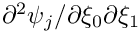2D: d2psids(i,0) =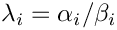d2psids(i,1) =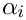d2psids(i,2) =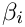3D: d2psids(i,0) =d2psids(i,1) =d2psids(i,2) =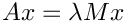d2psids(i,3) =d2psids(i,4) =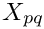d2psids(i,5) =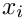. More...

virtual void d2shape_local_at_knot (const unsigned &ipt, Shape &psi, DShape &dpsids, DShape &d2psids) const
Return the geometric shape function and its first and second derivatives w.r.t. the local coordinates at the ipt-th integration point. Numbering: 1D: d2psids(i,0) =2D: d2psids(i,0) =d2psids(i,1) =d2psids(i,2) =3D: d2psids(i,0) =d2psids(i,1) =d2psids(i,2) =d2psids(i,3) =d2psids(i,4) =d2psids(i,5) =. More...

virtual double J_eulerian (const Vector< double > &s) const
Return the Jacobian of mapping from local to global coordinates at local position s. More...

virtual double J_eulerian_at_knot (const unsigned &ipt) const
Return the Jacobian of the mapping from local to global coordinates at the ipt-th integration point. More...

void check_J_eulerian_at_knots (bool &passed) const
Check that Jacobian of mapping between local and Eulerian coordinates at all integration points is positive. More...

void check_jacobian (const double &jacobian) const
Helper function used to check for singular or negative Jacobians in the transform from local to global or Lagrangian coordinates. More...

double dshape_eulerian (const Vector< double > &s, Shape &psi, DShape &dpsidx) const
Compute the geometric shape functions and also first derivatives w.r.t. global coordinates at local coordinate s; Returns Jacobian of mapping from global to local coordinates. More...

virtual double dshape_eulerian_at_knot (const unsigned &ipt, Shape &psi, DShape &dpsidx) const
Return the geometric shape functions and also first derivatives w.r.t. global coordinates at the ipt-th integration point. More...

virtual double dshape_eulerian_at_knot (const unsigned &ipt, Shape &psi, DShape &dpsi, DenseMatrix< double > &djacobian_dX, RankFourTensor< double > &d_dpsidx_dX) const
Compute the geometric shape functions (psi) and first derivatives w.r.t. global coordinates (dpsidx) at the ipt-th integration point. Return the determinant of the jacobian of the mapping (detJ). Additionally calculate the derivatives of both "detJ" and "dpsidx" w.r.t. the nodal coordinates. More...

double d2shape_eulerian (const Vector< double > &s, Shape &psi, DShape &dpsidx, DShape &d2psidx) const
Compute the geometric shape functions and also first and second derivatives w.r.t. global coordinates at local coordinate s; Returns Jacobian of mapping from global to local coordinates. Numbering: 1D: d2psidx(i,0) =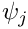2D: d2psidx(i,0) =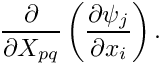d2psidx(i,1) =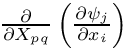d2psidx(i,2) =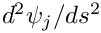3D: d2psidx(i,0) =d2psidx(i,1) =d2psidx(i,2) =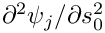d2psidx(i,3) =d2psidx(i,4) =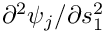d2psidx(i,5) =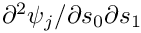. More...

virtual double d2shape_eulerian_at_knot (const unsigned &ipt, Shape &psi, DShape &dpsidx, DShape &d2psidx) const
Return the geometric shape functions and also first and second derivatives w.r.t. global coordinates at ipt-th integration point. Numbering: 1D: d2psidx(i,0) =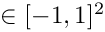2D: d2psidx(i,0) =d2psidx(i,1) =d2psidx(i,2) =3D: d2psidx(i,0) =d2psidx(i,1) =d2psidx(i,2) =d2psidx(i,3) =d2psidx(i,4) =d2psidx(i,5) =. More...

virtual void assign_nodal_local_eqn_numbers (const bool &store_local_dof_pt)
Assign the local equation numbers for Data stored at the nodes Virtual so that it can be overloaded by RefineableFiniteElements. If the boolean is true then the pointers to the degrees of freedom associated with each equation number are stored in Dof_pt. More...

virtual void describe_local_dofs (std::ostream &out, const std::string &current_string) const
Function to describe the local dofs of the element[s]. The ostream specifies the output stream to which the description is written; the string stores the currently assembled output that is ultimately written to the output stream by Data::describe_dofs(...); it is typically built up incrementally as we descend through the call hierarchy of this function when called from Problem::describe_dofs(...) More...

virtual void describe_nodal_local_dofs (std::ostream &out, const std::string &current_string) const
Function to describe the local dofs of the element[s]. The ostream specifies the output stream to which the description is written; the string stores the currently assembled output that is ultimately written to the output stream by Data::describe_dofs(...); it is typically built up incrementally as we descend through the call hierarchy of this function when called from Problem::describe_dofs(...) More...

virtual void assign_all_generic_local_eqn_numbers (const bool &store_local_dof_pt)
Overloaded version of the calculation of the local equation numbers. If the boolean argument is true then pointers to the degrees of freedom associated with each equation number are stored locally in the array Dof_pt. More...

Node *& node_pt (const unsigned &n)
Return a pointer to the local node n. More...

Node *const & node_pt (const unsigned &n) const
Return a pointer to the local node n (const version) More...

unsigned nnode () const
Return the number of nodes. More...

virtual unsigned nnode_1d () const
Return the number of nodes along one edge of the element Default is to return zero — must be overloaded by geometric elements. More...

double raw_nodal_position (const unsigned &n, const unsigned &i) const
Return the i-th coordinate at local node n. Do not use the hanging node representation. NOTE: Moved to cc file because of a possible compiler bug in gcc (yes, really!). The move to the cc file avoids inlining which appears to cause problems (only) when compiled with gcc and -O3; offensive "illegal read" is in optimised-out section of code and data that is allegedly illegal is readily readable (by other means) just before this function is called so I can't really see how we could possibly be responsible for this... More...

double raw_nodal_position (const unsigned &t, const unsigned &n, const unsigned &i) const
Return the i-th coordinate at local node n, at time level t (t=0: present; t>0: previous time level). Do not use the hanging node representation. More...

double raw_dnodal_position_dt (const unsigned &n, const unsigned &i) const
Return the i-th component of nodal velocity: dx/dt at local node n. Do not use the hanging node representation. More...

double raw_dnodal_position_dt (const unsigned &n, const unsigned &j, const unsigned &i) const
Return the i-th component of j-th derivative of nodal position: d^jx/dt^j at node n. Do not use the hanging node representation. More...

double raw_nodal_position_gen (const unsigned &n, const unsigned &k, const unsigned &i) const
Return the value of the k-th type of the i-th positional variable at the local node n. Do not use the hanging node representation. More...

double raw_nodal_position_gen (const unsigned &t, const unsigned &n, const unsigned &k, const unsigned &i) const
Return the generalised nodal position (type k, i-th variable) at previous timesteps at local node n. Do not use the hanging node representation. More...

double raw_dnodal_position_gen_dt (const unsigned &n, const unsigned &k, const unsigned &i) const
i-th component of time derivative (velocity) of the generalised position, dx(k,i)/dt at local node n. ‘Type’: k; Coordinate direction: i. Do not use the hanging node representation. More...

double raw_dnodal_position_gen_dt (const unsigned &j, const unsigned &n, const unsigned &k, const unsigned &i) const
i-th component of j-th time derivative of the generalised position, dx(k,i)/dt at local node n. ‘Type’: k; Coordinate direction: i. Do not use the hanging node representation. More...

double nodal_position (const unsigned &n, const unsigned &i) const
Return the i-th coordinate at local node n. If the node is hanging, the appropriate interpolation is handled by the position function in the Node class. More...

double nodal_position (const unsigned &t, const unsigned &n, const unsigned &i) const
Return the i-th coordinate at local node n, at time level t (t=0: present; t>0: previous time level) Returns suitably interpolated version for hanging nodes. More...

double dnodal_position_dt (const unsigned &n, const unsigned &i) const
Return the i-th component of nodal velocity: dx/dt at local node n. More...

double dnodal_position_dt (const unsigned &n, const unsigned &j, const unsigned &i) const
Return the i-th component of j-th derivative of nodal position: d^jx/dt^j at node n. More...

double nodal_position_gen (const unsigned &n, const unsigned &k, const unsigned &i) const
Return the value of the k-th type of the i-th positional variable at the local node n. More...

double nodal_position_gen (const unsigned &t, const unsigned &n, const unsigned &k, const unsigned &i) const
Return the generalised nodal position (type k, i-th variable) at previous timesteps at local node n. More...

double dnodal_position_gen_dt (const unsigned &n, const unsigned &k, const unsigned &i) const
i-th component of time derivative (velocity) of the generalised position, dx(k,i)/dt at local node n. ‘Type’: k; Coordinate direction: i. More...

double dnodal_position_gen_dt (const unsigned &j, const unsigned &n, const unsigned &k, const unsigned &i) const
i-th component of j-th time derivative of the generalised position, dx(k,i)/dt at local node n. ‘Type’: k; Coordinate direction: i. More...

virtual void get_dresidual_dnodal_coordinates (RankThreeTensor< double > &dresidual_dnodal_coordinates)
Compute derivatives of elemental residual vector with respect to nodal coordinates. Default implementation by FD can be overwritten for specific elements. dresidual_dnodal_coordinates(l,i,j) = d res(l) / dX_{ij}. More...

virtual void disable_ALE ()
This is an empty function that establishes a uniform interface for all (derived) elements that involve time-derivatives. Such elements are/should be implemented in ALE form to allow mesh motions. The additional expense associated with the computation of the mesh velocities is, of course, superfluous if the elements are used in problems in which the mesh is stationary. This function should therefore be overloaded in all derived elements that are formulated in ALE form to suppress the computation of the mesh velocities. The user disables the ALE functionality at his/her own risk! If the mesh does move after all, then the results will be wrong. Here we simply issue a warning message stating that the empty function has been called. More...

virtual void enable_ALE ()
(Re-)enable ALE, i.e. take possible mesh motion into account when evaluating the time-derivative. This function is empty and simply establishes a common interface for all derived elements that are formulated in ALE form. More...

virtual unsigned required_nvalue (const unsigned &n) const
Number of values that must be stored at local node n by the element. The default is 0, until over-ridden by a particular element. For example, a Poisson equation requires only one value to be stored at each node; 2D Navier–Stokes equations require two values (velocity components) to be stored at each Node (provided that the pressure interpolation is discontinuous). More...

unsigned nnodal_position_type () const
Return the number of coordinate types that the element requires to interpolate the geometry between the nodes. For Lagrange elements it is 1. More...

bool has_hanging_nodes () const
Return boolean to indicate if any of the element's nodes are geometrically hanging. More...

unsigned nodal_dimension () const
Return the required Eulerian dimension of the nodes in this element. More...

virtual unsigned nvertex_node () const
Return the number of vertex nodes in this element. Broken virtual function in "pure" finite elements. More...

virtual Nodevertex_node_pt (const unsigned &j) const
Pointer to the j-th vertex node in the element. Broken virtual function in "pure" finite elements. More...

virtual Nodeconstruct_node (const unsigned &n)
Construct the local node n and return a pointer to the newly created node object. More...

virtual Nodeconstruct_node (const unsigned &n, TimeStepper *const &time_stepper_pt)
Construct the local node n, including storage for history values required by timestepper, and return a pointer to the newly created node object. More...

virtual Nodeconstruct_boundary_node (const unsigned &n)
Construct the local node n as a boundary node; that is a node that MAY be placed on a mesh boundary and return a pointer to the newly created node object. More...

virtual Nodeconstruct_boundary_node (const unsigned &n, TimeStepper *const &time_stepper_pt)
Construct the local node n, including storage for history values required by timestepper, as a boundary node; that is a node that MAY be placed on a mesh boundary and return a pointer to the newly created node object. More...

int get_node_number (Node *const &node_pt) const
Return the number of the node *node_pt if this node is in the element, else return -1;. More...

virtual Nodeget_node_at_local_coordinate (const Vector< double > &s) const
If there is a node at this local coordinate, return the pointer to the node. More...

double raw_nodal_value (const unsigned &n, const unsigned &i) const
Return the i-th value stored at local node n but do NOT take hanging nodes into account. More...

double raw_nodal_value (const unsigned &t, const unsigned &n, const unsigned &i) const
Return the i-th value stored at local node n, at time level t (t=0: present; t>0 previous timesteps), but do NOT take hanging nodes into account. More...

double nodal_value (const unsigned &n, const unsigned &i) const
Return the i-th value stored at local node n. Produces suitably interpolated values for hanging nodes. More...

double nodal_value (const unsigned &t, const unsigned &n, const unsigned &i) const
Return the i-th value stored at local node n, at time level t (t=0: present; t>0 previous timesteps). Produces suitably interpolated values for hanging nodes. More...

unsigned dim () const
Return the spatial dimension of the element, i.e. the number of local coordinates required to parametrise its geometry. More...

virtual ElementGeometry::ElementGeometry element_geometry () const
Return the geometry type of the element (either Q or T usually). More...

virtual double interpolated_x (const Vector< double > &s, const unsigned &i) const
Return FE interpolated coordinate x[i] at local coordinate s. More...

virtual double interpolated_x (const unsigned &t, const Vector< double > &s, const unsigned &i) const
Return FE interpolated coordinate x[i] at local coordinate s at previous timestep t (t=0: present; t>0: previous timestep) More...

virtual void interpolated_x (const Vector< double > &s, Vector< double > &x) const
Return FE interpolated position x[] at local coordinate s as Vector. More...

virtual void interpolated_x (const unsigned &t, const Vector< double > &s, Vector< double > &x) const
Return FE interpolated position x[] at local coordinate s at previous timestep t as Vector (t=0: present; t>0: previous timestep) More...

virtual double interpolated_dxdt (const Vector< double > &s, const unsigned &i, const unsigned &t)
Return t-th time-derivative of the i-th FE-interpolated Eulerian coordinate at local coordinate s. More...

virtual void interpolated_dxdt (const Vector< double > &s, const unsigned &t, Vector< double > &dxdt)
Compte t-th time-derivative of the FE-interpolated Eulerian coordinate vector at local coordinate s. More...

unsigned ngeom_data () const
A standard FiniteElement is fixed, so there are no geometric data when viewed in its GeomObject incarnation. More...

Datageom_data_pt (const unsigned &j)
A standard FiniteElement is fixed, so there are no geometric data when viewed in its GeomObject incarnation. More...

void position (const Vector< double > &zeta, Vector< double > &r) const
Return the parametrised position of the FiniteElement in its incarnation as a GeomObject, r(zeta). The position is given by the Eulerian coordinate and the intrinsic coordinate (zeta) is the local coordinate of the element (s). More...

void position (const unsigned &t, const Vector< double > &zeta, Vector< double > &r) const
Return the parametrised position of the FiniteElement in its GeomObject incarnation: r(zeta). The position is given by the Eulerian coordinate and the intrinsic coordinate (zeta) is the local coordinate of the element (s) This version of the function returns the position as a function of time t=0: current time; t>0: previous timestep. Works for t=0 but needs to be overloaded if genuine time-dependence is required. More...

void dposition_dt (const Vector< double > &zeta, const unsigned &t, Vector< double > &drdt)
Return the t-th time derivative of the parametrised position of the FiniteElement in its GeomObject incarnation:. Call the t-th time derivative of the FE-interpolated Eulerian coordinate. More...

virtual double zeta_nodal (const unsigned &n, const unsigned &k, const unsigned &i) const
Specify the values of the "global" intrinsic coordinate, zeta, of a compound geometric object (a mesh of elements) when the element is viewied as a sub-geometric object. The default assumption is that the element will be treated as a sub-geometric object in a bulk Mesh of other elements (geometric objects). The "global" coordinate of the compound geometric object is simply the Eulerian coordinate, x. The second default assumption is that the coordinate zeta will be stored at the nodes and interpolated using the shape functions of the element. This function returns the value of zeta stored at local node n, where k is the type of coordinate and i is the coordinate direction. The function is virtual so that it can be overloaded by different types of element: FaceElements and SolidFiniteElements. More...

void interpolated_zeta (const Vector< double > &s, Vector< double > &zeta) const
Calculate the interpolated value of zeta, the intrinsic coordinate of the element when viewed as a compound geometric object within a Mesh as a function of the local coordinate of the element, s. The default assumption is the zeta is interpolated using the shape functions of the element with the values given by zeta_nodal(). A MacroElement representation of the intrinsic coordinate parametrised by the local coordinate s is used if available. Choosing the MacroElement representation of zeta (Eulerian x by default) allows a correspondence to be established between elements on different Meshes covering the same curvilinear domain in cases where one element is much coarser than the other. More...

void locate_zeta (const Vector< double > &zeta, GeomObject *&geom_object_pt, Vector< double > &s, const bool &use_coordinate_as_initial_guess=false)
For a given value of zeta, the "global" intrinsic coordinate of a mesh of FiniteElements represented as a compound geometric object, find the local coordinate in this element that corresponds to the requested value of zeta. If zeta cannot be located in this element, geom_object_pt is set to NULL. If zeta is located in this element, we return its "this" pointer. By default don't use any value passed in to the local coordinate s as the initial guess in the Newton method. More...

virtual void node_update ()
Update the positions of all nodes in the element using each node update function. The default implementation may be overloaded so that more efficient versions can be written. More...

virtual void identify_field_data_for_interactions (std::set< std::pair< Data *, unsigned > > &paired_field_data)
The purpose of this function is to identify all possible Data that can affect the fields interpolated by the FiniteElement. The information will typically be used in interaction problems in which the FiniteElement provides a forcing term for an ElementWithExternalElement. The Data must be provided as paired_load data containing. More...

virtual void identify_geometric_data (std::set< Data * > &geometric_data_pt)
The purpose of this function is to identify all Data objects that affect the elements' geometry. This function is implemented as an empty virtual function since it can only be implemented in conjunction with a node-update strategy. A specific implementation is provided in the ElementWithMovingNodes class. More...

virtual double s_min () const
Min value of local coordinate. More...

virtual double s_max () const
Max. value of local coordinate. More...

double size () const
Calculate the size of the element (length, area, volume,...) in Eulerian computational coordinates. Use suitably overloaded compute_physical_size() function to compute the actual size (taking into account factors such as 2pi or radii the integrand) – such function can only be implemented on an equation-by-equation basis. More...

virtual double compute_physical_size () const
Broken virtual function to compute the actual size (taking into account factors such as 2pi or radii the integrand) – such function can only be implemented on an equation-by-equation basis. More...

virtual void point_output_data (const Vector< double > &s, Vector< double > &data)
Virtual function to write the double precision numbers that appear in a single line of output into the data vector. Empty virtual, can be overloaded for specific elements; used e.g. by LineVisualiser. More...

void point_output (std::ostream &outfile, const Vector< double > &s)
Output solution (as defined by point_output_data()) at local cordinates s. More...

virtual unsigned nplot_points_paraview (const unsigned &nplot) const
Return the number of actual plot points for paraview plot with parameter nplot. Broken virtual; can be overloaded in specific elements. More...

virtual unsigned nsub_elements_paraview (const unsigned &nplot) const
Return the number of local sub-elements for paraview plot with parameter nplot. Broken virtual; can be overloaded in specific elements. More...

void output_paraview (std::ofstream &file_out, const unsigned &nplot) const
Paraview output – this outputs the coordinates at the plot points (for parameter nplot) to specified output file. More...

virtual void write_paraview_output_offset_information (std::ofstream &file_out, const unsigned &nplot, unsigned &counter) const
Fill in the offset information for paraview plot. Broken virtual. Needs to be implemented for each new geometric element type; see http://www.vtk.org/VTK/img/file-formats.pdf. More...

virtual void write_paraview_type (std::ofstream &file_out, const unsigned &nplot) const
Return the paraview element type. Broken virtual. Needs to be implemented for each new geometric element type; see http://www.vtk.org/VTK/img/file-formats.pdf. More...

virtual void write_paraview_offsets (std::ofstream &file_out, const unsigned &nplot, unsigned &offset_sum) const
Return the offsets for the paraview sub-elements. Broken virtual. Needs to be implemented for each new geometric element type; see http://www.vtk.org/VTK/img/file-formats.pdf. More...

virtual unsigned nscalar_paraview () const
Number of scalars/fields output by this element. Broken virtual. Needs to be implemented for each new specific element type. More...

virtual void scalar_value_paraview (std::ofstream &file_out, const unsigned &i, const unsigned &nplot) const
Write values of the i-th scalar field at the plot points. Broken virtual. Needs to be implemented for each new specific element type. More...

virtual void scalar_value_fct_paraview (std::ofstream &file_out, const unsigned &i, const unsigned &nplot, FiniteElement::SteadyExactSolutionFctPt exact_soln_pt) const
Write values of the i-th scalar field at the plot points. Broken virtual. Needs to be implemented for each new specific element type. More...

virtual void scalar_value_fct_paraview (std::ofstream &file_out, const unsigned &i, const unsigned &nplot, const double &time, FiniteElement::UnsteadyExactSolutionFctPt exact_soln_pt) const
Write values of the i-th scalar field at the plot points. Broken virtual. Needs to be implemented for each new specific element type. More...

virtual std::string scalar_name_paraview (const unsigned &i) const
Name of the i-th scalar field. Default implementation returns V1 for the first one, V2 for the second etc. Can (should!) be overloaded with more meaningful names in specific elements. More...

virtual void output (std::ostream &outfile)
Output the element data — typically the values at the nodes in a format suitable for post-processing. More...

virtual void output (std::ostream &outfile, const unsigned &n_plot)
Output the element data — pass (some measure of) the number of plot points per element. More...

virtual void output (const unsigned &t, std::ostream &outfile, const unsigned &n_plot) const
Output the element data at time step t. This is const because it is newly added and so can be done easily. Really all the output(...) functions should be const! More...

virtual void output (FILE *file_pt)
Output the element data — typically the values at the nodes in a format suitable for post-processing. (C style output) More...

virtual void output (FILE *file_pt, const unsigned &n_plot)
Output the element data — pass (some measure of) the number of plot points per element (C style output) More...

virtual void output_fct (std::ostream &outfile, const unsigned &n_plot, FiniteElement::SteadyExactSolutionFctPt exact_soln_pt)
Output an exact solution over the element. More...

virtual void output_fct (std::ostream &outfile, const unsigned &n_plot, const double &time, FiniteElement::UnsteadyExactSolutionFctPt exact_soln_pt)
Output a time-dependent exact solution over the element. More...

virtual void output_fct (std::ostream &outfile, const unsigned &n_plot, const double &time, const SolutionFunctorBase &exact_soln) const
Output a time-dependent exact solution over the element. More...

virtual void get_s_plot (const unsigned &i, const unsigned &nplot, Vector< double > &s, const bool &shifted_to_interior=false) const
Get cector of local coordinates of plot point i (when plotting nplot points in each "coordinate direction"). Generally these plot points will be uniformly spaced across the element. The optional final boolean flag (default: false) allows them to be shifted inwards to avoid duplication of plot point points between elements – useful when they are used in locate_zeta, say. More...

virtual std::string tecplot_zone_string (const unsigned &nplot) const
Return string for tecplot zone header (when plotting nplot points in each "coordinate direction") More...

virtual void write_tecplot_zone_footer (std::ostream &outfile, const unsigned &nplot) const
Add tecplot zone "footer" to output stream (when plotting nplot points in each "coordinate direction"). Empty by default – can be used, e.g., to add FE connectivity lists to elements that need it. More...

virtual void write_tecplot_zone_footer (FILE *file_pt, const unsigned &nplot) const
Add tecplot zone "footer" to C-style output. (when plotting nplot points in each "coordinate direction"). Empty by default – can be used, e.g., to add FE connectivity lists to elements that need it. More...

virtual unsigned nplot_points (const unsigned &nplot) const
Return total number of plot points (when plotting nplot points in each "coordinate direction") More...

virtual void compute_error (FiniteElement::SteadyExactSolutionFctPt exact_soln_pt, double &error, double &norm)
Calculate the norm of the error and that of the exact solution. More...

virtual void compute_error (FiniteElement::UnsteadyExactSolutionFctPt exact_soln_pt, const double &time, double &error, double &norm)
Calculate the norm of the error and that of the exact solution. More...

virtual void compute_error (FiniteElement::SteadyExactSolutionFctPt exact_soln_pt, Vector< double > &error, Vector< double > &norm)
Given the exact solution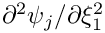this function calculates the norm of the error and that of the exact solution. Version with vectors of norms and errors so that different variables' norms and errors can be returned individually. More...

virtual void compute_error (FiniteElement::UnsteadyExactSolutionFctPt exact_soln_pt, const double &time, Vector< double > &error, Vector< double > &norm)
Given the exact solutionthis function calculates the norm of the error and that of the exact solution. Version with vectors of norms and errors so that different variables' norms and errors can be returned individually. More...

virtual void compute_error (std::ostream &outfile, FiniteElement::SteadyExactSolutionFctPt exact_soln_pt, double &error, double &norm)
Plot the error when compared against a given exact solution. Also calculates the norm of the error and that of the exact solution. More...

virtual void compute_error (std::ostream &outfile, FiniteElement::UnsteadyExactSolutionFctPt exact_soln_pt, const double &time, double &error, double &norm)
Plot the error when compared against a given time-dependent exact solution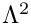. Also calculates the norm of the error and that of the exact solution. More...

virtual void compute_error (std::ostream &outfile, FiniteElement::SteadyExactSolutionFctPt exact_soln_pt, Vector< double > &error, Vector< double > &norm)
Plot the error when compared against a given exact solution. Also calculates the norm of the error and that of the exact solution. The version with vectors of norms and errors so that different variables' norms and errors can be returned individually. More...

virtual void compute_error (std::ostream &outfile, FiniteElement::UnsteadyExactSolutionFctPt exact_soln_pt, const double &time, Vector< double > &error, Vector< double > &norm)
Plot the error when compared against a given time-dependent exact solution. Also calculates the norm of the error and that of the exact solution. The version with vectors of norms and errors so that different variables' norms and errors can be returned individually. More...

virtual void compute_abs_error (std::ostream &outfile, FiniteElement::SteadyExactSolutionFctPt exact_soln_pt, double &error)
Plot the error when compared against a given exact solution. Also calculates the maximum absolute error. More...

void integrate_fct (FiniteElement::SteadyExactSolutionFctPt integrand_fct_pt, Vector< double > &integral)
Evaluate integral of a Vector-valued functionover the element. More...

void integrate_fct (FiniteElement::UnsteadyExactSolutionFctPt integrand_fct_pt, const double &time, Vector< double > &integral)
Evaluate integral of a Vector-valued, time-dependent functionover the element. More...

virtual void build_face_element (const int &face_index, FaceElement *face_element_pt)
Function for building a lower dimensional FaceElement on the specified face of the FiniteElement. The arguments are the index of the face, an integer whose value depends on the particular element type, and a pointer to the FaceElement. More...

virtual unsigned self_test ()
Self-test: Check inversion of element & do self-test for GeneralisedElement. Return 0 if OK. More...

virtual unsigned get_bulk_node_number (const int &face_index, const unsigned &i) const
Get the number of the ith node on face face_index (in the bulk node vector). More...

virtual int face_outer_unit_normal_sign (const int &face_index) const
Get the sign of the outer unit normal on the face given by face_index. More...

virtual unsigned nnode_on_face () const

void face_node_number_error_check (const unsigned &i) const
Range check for face node numbers. More...

virtual CoordinateMappingFctPt face_to_bulk_coordinate_fct_pt (const int &face_index) const
Get a pointer to the function mapping face coordinates to bulk coordinates. More...

virtual BulkCoordinateDerivativesFctPt bulk_coordinate_derivatives_fct_pt (const int &face_index) const
Get a pointer to the derivative of the mapping from face to bulk coordinates. More...Public Member Functions inherited from oomph::GeneralisedElement
GeneralisedElement() GeneralisedElement (const GeneralisedElement &)=delete
Constructor: Initialise all pointers and all values to zero. More...

void operator= (const GeneralisedElement &)=delete
Broken assignment operator. More...

Data *& internal_data_pt (const unsigned &i)
Return a pointer to i-th internal data object. More...

Data *const & internal_data_pt (const unsigned &i) const
Return a pointer to i-th internal data object (const version) More...

Data *& external_data_pt (const unsigned &i)
Return a pointer to i-th external data object. More...

Data *const & external_data_pt (const unsigned &i) const
Return a pointer to i-th external data object (const version) More...

unsigned long eqn_number (const unsigned &ieqn_local) const
Return the global equation number corresponding to the ieqn_local-th local equation number. More...

int local_eqn_number (const unsigned long &ieqn_global) const
Return the local equation number corresponding to the ieqn_global-th global equation number. Returns minus one (-1) if there is no local degree of freedom corresponding to the chosen global equation number. More...

unsigned add_external_data (Data *const &data_pt, const bool &fd=true)
Add a (pointer to an) external data object to the element and return its index (i.e. the index required to obtain it from the access function external_data_pt(...). The optional boolean flag indicates whether the data should be included in the general finite-difference loop when calculating the jacobian. The default value is true, i.e. the data will be included in the finite-differencing. More...

bool external_data_fd (const unsigned &i) const
Return the status of the boolean flag indicating whether the external data is included in the finite difference loop. More...

void exclude_external_data_fd (const unsigned &i)
Set the boolean flag to exclude the external datum from the the finite difference loop when computing the jacobian matrix. More...

void include_external_data_fd (const unsigned &i)
Set the boolean flag to include the external datum in the the finite difference loop when computing the jacobian matrix. More...

void flush_external_data ()
Flush all external data. More...

void flush_external_data (Data *const &data_pt)
Flush the object addressed by data_pt from the external data array. More...

unsigned ninternal_data () const
Return the number of internal data objects. More...

unsigned nexternal_data () const
Return the number of external data objects. More...

unsigned ndof () const
Return the number of equations/dofs in the element. More...

void dof_vector (const unsigned &t, Vector< double > &dof)
Return the vector of dof values at time level t. More...

void dof_pt_vector (Vector< double * > &dof_pt)
Return the vector of pointers to dof values. More...

void set_internal_data_time_stepper (const unsigned &i, TimeStepper *const &time_stepper_pt, const bool &preserve_existing_data)
Set the timestepper associated with the i-th internal data object. More...

void assign_internal_eqn_numbers (unsigned long &global_number, Vector< double * > &Dof_pt)
Assign the global equation numbers to the internal Data. The arguments are the current highest global equation number (which will be incremented) and a Vector of pointers to the global variables (to which any unpinned values in the internal Data are added). More...

void describe_dofs (std::ostream &out, const std::string &current_string) const
Function to describe the dofs of the element. The ostream specifies the output stream to which the description is written; the string stores the currently assembled output that is ultimately written to the output stream by Data::describe_dofs(...); it is typically built up incrementally as we descend through the call hierarchy of this function when called from Problem::describe_dofs(...) More...

void add_internal_value_pt_to_map (std::map< unsigned, double * > &map_of_value_pt)
Add pointers to the internal data values to map indexed by the global equation number. More...

void add_internal_data_values_to_vector (Vector< double > &vector_of_values)
Add all internal data and time history values to the vector in the internal storage order. More...

void read_internal_data_values_from_vector (const Vector< double > &vector_of_values, unsigned &index)
Read all internal data and time history values from the vector starting from index. On return the index will be set to the value at the end of the data that has been read in. More...

void add_internal_eqn_numbers_to_vector (Vector< long > &vector_of_eqn_numbers)
Add all equation numbers associated with internal data to the vector in the internal storage order. More...

void read_internal_eqn_numbers_from_vector (const Vector< long > &vector_of_eqn_numbers, unsigned &index)
Read all equation numbers associated with internal data from the vector starting from index. On return the index will be set to the value at the end of the data that has been read in. More...

virtual void assign_local_eqn_numbers (const bool &store_local_dof_pt)
Setup the arrays of local equation numbers for the element. If the optional boolean argument is true, then pointers to the associated degrees of freedom are stored locally in the array Dof_pt. More...

virtual void complete_setup_of_dependencies ()
Complete the setup of any additional dependencies that the element may have. Empty virtual function that may be overloaded for specific derived elements. Used, e.g., for elements with algebraic node update functions to determine the "geometric Data", i.e. the Data that affects the element's shape. This function is called (for all elements) at the very beginning of the equation numbering procedure to ensure that all dependencies are accounted for. More...

virtual void get_residuals (Vector< double > &residuals)
Calculate the vector of residuals of the equations in the element. By default initialise the vector to zero and then call the fill_in_contribution_to_residuals() function. Note that this entire function can be overloaded if desired. More...

virtual void get_jacobian (Vector< double > &residuals, DenseMatrix< double > &jacobian)
Calculate the elemental Jacobian matrix "d equation / d variable". More...

virtual void get_mass_matrix (Vector< double > &residuals, DenseMatrix< double > &mass_matrix)
Calculate the residuals and the elemental "mass" matrix, the matrix that multiplies the time derivative terms in a problem. More...

virtual void get_jacobian_and_mass_matrix (Vector< double > &residuals, DenseMatrix< double > &jacobian, DenseMatrix< double > &mass_matrix)
Calculate the residuals and jacobian and elemental "mass" matrix, the matrix that multiplies the time derivative terms. More...

virtual void get_dresiduals_dparameter (double *const &parameter_pt, Vector< double > &dres_dparam)
Calculate the derivatives of the residuals with respect to a parameter. More...

virtual void get_djacobian_dparameter (double *const &parameter_pt, Vector< double > &dres_dparam, DenseMatrix< double > &djac_dparam)
Calculate the derivatives of the elemental Jacobian matrix and residuals with respect to a parameter. More...

virtual void get_djacobian_and_dmass_matrix_dparameter (double *const &parameter_pt, Vector< double > &dres_dparam, DenseMatrix< double > &djac_dparam, DenseMatrix< double > &dmass_matrix_dparam)
Calculate the derivatives of the elemental Jacobian matrix mass matrix and residuals with respect to a parameter. More...

virtual void get_hessian_vector_products (Vector< double > const &Y, DenseMatrix< double > const &C, DenseMatrix< double > &product)
Calculate the product of the Hessian (derivative of Jacobian with respect to all variables) an eigenvector, Y, and other specified vectors, C (d(J_{ij})/d u_{k}) Y_{j} C_{k}. More...

virtual void get_inner_products (Vector< std::pair< unsigned, unsigned > > const &history_index, Vector< double > &inner_product)
Return the vector of inner product of the given pairs of history values. More...

virtual void get_inner_product_vectors (Vector< unsigned > const &history_index, Vector< Vector< double > > &inner_product_vector)
Compute the vectors that when taken as a dot product with other history values give the inner product over the element. More...

virtual void compute_norm (Vector< double > &norm)
Compute norm of solution – broken virtual can be overloaded by element writer to implement whatever norm is desired for the specific element. More...

virtual void compute_norm (double &norm)
Compute norm of solution – broken virtual can be overloaded by element writer to implement whatever norm is desired for the specific element. More...

void set_halo (const unsigned &non_halo_proc_ID)
Label the element as halo and specify processor that holds non-halo counterpart. More...

void set_nonhalo ()
Label the element as not being a halo. More...

bool is_halo () const
Is this element a halo? More...

int non_halo_proc_ID ()
ID of processor ID that holds non-halo counterpart of halo element; negative if not a halo. More...

void set_must_be_kept_as_halo ()
Insist that this element be kept as a halo element during a distribute? More...

void unset_must_be_kept_as_halo ()
Do not insist that this element be kept as a halo element during distribution. More...

bool must_be_kept_as_halo () const
Test whether the element must be kept as a halo element. More...

virtual unsigned ndof_types () const
The number of types of degrees of freedom in this element are sub-divided into. More...

virtual void get_dof_numbers_for_unknowns (std::list< std::pair< unsigned long, unsigned > > &dof_lookup_list) const
Create a list of pairs for the unknowns that this element is "in charge of" – ignore any unknowns associated with external Data. The first entry in each pair must contain the global equation number of the unknown, while the second one contains the number of the DOF type that this unknown is associated with. (The function can obviously only be called if the equation numbering scheme has been set up.) More...Public Member Functions inherited from oomph::GeomObject
GeomObject ()
Default constructor. More...

GeomObject (const unsigned &ndim)
Constructor: Pass dimension of geometric object (# of Eulerian coords = # of Lagrangian coords; no time history available/needed) More...

GeomObject (const unsigned &nlagrangian, const unsigned &ndim)
Constructor: pass # of Eulerian and Lagrangian coordinates. No time history available/needed. More...

GeomObject (const unsigned &nlagrangian, const unsigned &ndim, TimeStepper *time_stepper_pt)
Constructor: pass # of Eulerian and Lagrangian coordinates and pointer to time-stepper which is used to handle the position at previous timesteps and allows the evaluation of veloc/acceleration etc. in cases where the GeomData varies with time. More...

GeomObject (const GeomObject &dummy)=delete
Broken copy constructor. More...

void operator= (const GeomObject &)=delete
Broken assignment operator. More...

virtual ~GeomObject ()
(Empty) destructor More...

unsigned nlagrangian () const
Access function to # of Lagrangian coordinates. More...

unsigned ndim () const
Access function to # of Eulerian coordinates. More...

void set_nlagrangian_and_ndim (const unsigned &n_lagrangian, const unsigned &n_dim)
Set # of Lagrangian and Eulerian coordinates. More...

TimeStepper *& time_stepper_pt ()
Access function for pointer to time stepper: Null if object is not time-dependent. More...

TimeSteppertime_stepper_pt () const
Access function for pointer to time stepper: Null if object is not time-dependent. Const version. More...

virtual void position (const double &t, const Vector< double > &zeta, Vector< double > &r) const
Parametrised position on object: r(zeta). Evaluated at the continuous time value, t. More...

virtual void dposition (const Vector< double > &zeta, DenseMatrix< double > &drdzeta) const
Derivative of position Vector w.r.t. to coordinates: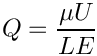= drdzeta(alpha,i). Evaluated at current time. More...

virtual void d2position (const Vector< double > &zeta, RankThreeTensor< double > &ddrdzeta) const
2nd derivative of position Vector w.r.t. to coordinates: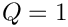= ddrdzeta(alpha,beta,i). Evaluated at current time. More...

virtual void d2position (const Vector< double > &zeta, Vector< double > &r, DenseMatrix< double > &drdzeta, RankThreeTensor< double > &ddrdzeta) const
Posn Vector and its 1st & 2nd derivatives w.r.t. to coordinates:= drdzeta(alpha,i).= ddrdzeta(alpha,beta,i). Evaluated at current time. More...

## Additional Inherited MembersPublic Types inherited from oomph::FiniteElement
typedef void(* SteadyExactSolutionFctPt) (const Vector< double > &, Vector< double > &)
Function pointer for function that computes vector-valued steady "exact solution"as. More...

typedef void(* UnsteadyExactSolutionFctPt) (const double &, const Vector< double > &, Vector< double > &)
Function pointer for function that computes Vector-valued time-dependent functionas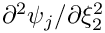. More...Static Public Attributes inherited from oomph::FiniteElement
static double Tolerance_for_singular_jacobian = 1.0e-16
Tolerance below which the jacobian is considered singular. More...

static bool Accept_negative_jacobian = false
Boolean that if set to true allows a negative jacobian in the transform between global and local coordinates (negative surface area = left-handed coordinate system). More...

static bool Suppress_output_while_checking_for_inverted_elements
Static boolean to suppress output while checking for inverted elements. More...Static Public Attributes inherited from oomph::GeneralisedElement
Static boolean to suppress warnings about repeated internal data. Defaults to false. More...

static bool Suppress_warning_about_repeated_external_data = true
Static boolean to suppress warnings about repeated external data. Defaults to true. More...

static double Default_fd_jacobian_step = 1.0e-8
Double used for the default finite difference step in elemental jacobian calculations. More...Protected Member Functions inherited from oomph::FiniteElement
virtual void assemble_local_to_eulerian_jacobian (const DShape &dpsids, DenseMatrix< double > &jacobian) const
Assemble the jacobian matrix for the mapping from local to Eulerian coordinates, given the derivatives of the shape function w.r.t the local coordinates. More...

virtual void assemble_local_to_eulerian_jacobian2 (const DShape &d2psids, DenseMatrix< double > &jacobian2) const
Assemble the the "jacobian" matrix of second derivatives of the mapping from local to Eulerian coordinates, given the second derivatives of the shape functions w.r.t. local coordinates. More...

virtual void assemble_eulerian_base_vectors (const DShape &dpsids, DenseMatrix< double > &interpolated_G) const
Assemble the covariant Eulerian base vectors, assuming that the derivatives of the shape functions with respect to the local coordinates have already been constructed. More...

template<unsigned DIM>
double invert_jacobian (const DenseMatrix< double > &jacobian, DenseMatrix< double > &inverse_jacobian) const
Take the matrix passed as jacobian and return its inverse in inverse_jacobian. This function is templated by the dimension of the element because matrix inversion cannot be written efficiently in a generic manner. More...

virtual double invert_jacobian_mapping (const DenseMatrix< double > &jacobian, DenseMatrix< double > &inverse_jacobian) const
A template-free interface that takes the matrix passed as jacobian and return its inverse in inverse_jacobian. By default the function will use the dimension of the element to call the correct invert_jacobian(..) function. This should be overloaded for efficiency (removal of a switch statement) in specific elements. More...

virtual double local_to_eulerian_mapping (const DShape &dpsids, DenseMatrix< double > &jacobian, DenseMatrix< double > &inverse_jacobian) const
Calculate the mapping from local to Eulerian coordinates, given the derivatives of the shape functions w.r.t. local coordinates. Returns the determinant of the jacobian, the jacobian and inverse jacobian. More...

double local_to_eulerian_mapping (const DShape &dpsids, DenseMatrix< double > &inverse_jacobian) const
Calculate the mapping from local to Eulerian coordinates, given the derivatives of the shape functions w.r.t. local coordinates, Return only the determinant of the jacobian and the inverse of the mapping (ds/dx). More...

virtual double local_to_eulerian_mapping_diagonal (const DShape &dpsids, DenseMatrix< double > &jacobian, DenseMatrix< double > &inverse_jacobian) const
Calculate the mapping from local to Eulerian coordinates given the derivatives of the shape functions w.r.t the local coordinates. assuming that the coordinates are aligned in the direction of the local coordinates, i.e. there are no cross terms and the jacobian is diagonal. This function returns the determinant of the jacobian, the jacobian and the inverse jacobian. More...

virtual void dJ_eulerian_dnodal_coordinates (const DenseMatrix< double > &jacobian, const DShape &dpsids, DenseMatrix< double > &djacobian_dX) const
A template-free interface that calculates the derivative of the jacobian of a mapping with respect to the nodal coordinates X_ij. To do this it requires the jacobian matrix and the derivatives of the shape functions w.r.t. the local coordinates. By default the function will use the dimension of the element to call the correct dJ_eulerian_dnodal_coordinates_templated_helper(..) function. This should be overloaded for efficiency (removal of a switch statement) in specific elements. More...

template<unsigned DIM>
void dJ_eulerian_dnodal_coordinates_templated_helper (const DenseMatrix< double > &jacobian, const DShape &dpsids, DenseMatrix< double > &djacobian_dX) const
Calculate the derivative of the jacobian of a mapping with respect to the nodal coordinates X_ij using the jacobian matrix and the derivatives of the shape functions w.r.t. the local coordinates. This function is templated by the dimension of the element. More...

virtual void d_dshape_eulerian_dnodal_coordinates (const double &det_jacobian, const DenseMatrix< double > &jacobian, const DenseMatrix< double > &djacobian_dX, const DenseMatrix< double > &inverse_jacobian, const DShape &dpsids, RankFourTensor< double > &d_dpsidx_dX) const
A template-free interface that calculates the derivative w.r.t. the nodal coordinates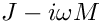of the derivative of the shape functions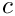w.r.t. the global eulerian coordinates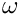. I.e. this function calculates. More...

template<unsigned DIM>
void d_dshape_eulerian_dnodal_coordinates_templated_helper (const double &det_jacobian, const DenseMatrix< double > &jacobian, const DenseMatrix< double > &djacobian_dX, const DenseMatrix< double > &inverse_jacobian, const DShape &dpsids, RankFourTensor< double > &d_dpsidx_dX) const
Calculate the derivative w.r.t. the nodal coordinatesof the derivative of the shape functions w.r.t. the global eulerian coordinates, using the determinant of the jacobian mapping, its derivative w.r.t. the nodal coordinates, the inverse jacobian and the derivatives of the shape functions w.r.t. the local coordinates. The result is returned as a tensor of rank four. Numbering: d_dpsidx_dX(p,q,j,i) =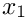This function is templated by the dimension of the element. More...

virtual void transform_derivatives (const DenseMatrix< double > &inverse_jacobian, DShape &dbasis) const
Convert derivative w.r.t.local coordinates to derivatives w.r.t the coordinates used to assemble the inverse_jacobian passed in the mapping. On entry, dbasis must contain the basis function derivatives w.r.t. the local coordinates; it will contain the derivatives w.r.t. the new coordinates on exit. This is virtual so that it may be overloaded if desired for efficiency reasons. More...

void transform_derivatives_diagonal (const DenseMatrix< double > &inverse_jacobian, DShape &dbasis) const
Convert derivative w.r.t local coordinates to derivatives w.r.t the coordinates used to assemble the inverse jacobian passed in the mapping, assuming that the coordinates are aligned in the direction of the local coordinates. On entry dbasis must contain the derivatives of the basis functions w.r.t. the local coordinates; it will contain the derivatives w.r.t. the new coordinates. are converted into the new using the mapping inverse_jacobian. More...

virtual void transform_second_derivatives (const DenseMatrix< double > &jacobian, const DenseMatrix< double > &inverse_jacobian, const DenseMatrix< double > &jacobian2, DShape &dbasis, DShape &d2basis) const
Convert derivatives and second derivatives w.r.t. local coordiantes to derivatives and second derivatives w.r.t. the coordinates used to assemble the jacobian, inverse jacobian and jacobian2 passed to the function. By default this function will call transform_second_derivatives_template<>(...) using the dimension of the element as the template parameter. It is virtual so that it can be overloaded by a specific element to save using a switch statement. Optionally, the element writer may wish to use the transform_second_derivatives_diagonal<>(...) function On entry dbasis and d2basis must contain the derivatives w.r.t. the local coordinates; on exit they will be the derivatives w.r.t. the transformed coordinates. More...

template<unsigned DIM>
void transform_second_derivatives_template (const DenseMatrix< double > &jacobian, const DenseMatrix< double > &inverse_jacobian, const DenseMatrix< double > &jacobian2, DShape &dbasis, DShape &d2basis) const
Convert derivatives and second derivatives w.r.t. local coordinates to derivatives and second derivatives w.r.t. the coordinates used to asssmble the jacobian, inverse jacobian and jacobian2 passed in the mapping. This is templated by dimension because the method of calculation varies significantly with the dimension. On entry dbasis and d2basis must contain the derivatives w.r.t. the local coordinates; on exit they will be the derivatives w.r.t. the transformed coordinates. More...

template<unsigned DIM>
void transform_second_derivatives_diagonal (const DenseMatrix< double > &jacobian, const DenseMatrix< double > &inverse_jacobian, const DenseMatrix< double > &jacobian2, DShape &dbasis, DShape &d2basis) const
Convert derivatives and second derivatives w.r.t. local coordinates to derivatives and second derivatives w.r.t. the coordinates used to asssmble the jacobian, inverse jacobian and jacobian2 passed in the mapping. This version of the function assumes that the local coordinates are aligned with the global coordinates, i.e. the jacobians are diagonal On entry dbasis and d2basis must contain the derivatives w.r.t. the local coordinates; on exit they will be the derivatives w.r.t. the transformed coordinates. More...

virtual void fill_in_jacobian_from_nodal_by_fd (Vector< double > &residuals, DenseMatrix< double > &jacobian)
Calculate the contributions to the jacobian from the nodal degrees of freedom using finite differences. This version of the function assumes that the residuals vector has already been calculated. More...

void fill_in_jacobian_from_nodal_by_fd (DenseMatrix< double > &jacobian)
Calculate the contributions to the jacobian from the nodal degrees of freedom using finite differences. This version computes the residuals vector before calculating the jacobian terms. More...

virtual void update_before_nodal_fd ()
Function that is called before the finite differencing of any nodal data. This may be overloaded to update any dependent data before finite differencing takes place. More...

virtual void reset_after_nodal_fd ()
Function that is call after the finite differencing of the nodal data. This may be overloaded to reset any dependent variables that may have changed during the finite differencing. More...

virtual void update_in_nodal_fd (const unsigned &i)
Function called within the finite difference loop for nodal data after a change in the i-th nodal value. More...

virtual void reset_in_nodal_fd (const unsigned &i)
Function called within the finite difference loop for nodal data after the i-th nodal values is reset. The default behaviour is to call the update function. More...

void fill_in_contribution_to_jacobian (Vector< double > &residuals, DenseMatrix< double > &jacobian)
Add the elemental contribution to the jacobian matrix. and the residuals vector. Note that this function will NOT initialise the residuals vector or the jacobian matrix. It must be called after the residuals vector and jacobian matrix have been initialised to zero. The default is to use finite differences to calculate the jacobian. More...

template<>
double invert_jacobian (const DenseMatrix< double > &jacobian, DenseMatrix< double > &inverse_jacobian) const
Zero-d specialisation of function to calculate inverse of jacobian mapping. More...

template<>
double invert_jacobian (const DenseMatrix< double > &jacobian, DenseMatrix< double > &inverse_jacobian) const
One-d specialisation of function to calculate inverse of jacobian mapping. More...

template<>
double invert_jacobian (const DenseMatrix< double > &jacobian, DenseMatrix< double > &inverse_jacobian) const
Two-d specialisation of function to calculate inverse of jacobian mapping. More...

template<>
double invert_jacobian (const DenseMatrix< double > &jacobian, DenseMatrix< double > &inverse_jacobian) const
Three-d specialisation of function to calculate inverse of jacobian mapping. More...

template<>
void dJ_eulerian_dnodal_coordinates_templated_helper (const DenseMatrix< double > &jacobian, const DShape &dpsids, DenseMatrix< double > &djacobian_dX) const
Zero-d specialisation of function to calculate the derivative of the jacobian of a mapping with respect to the nodal coordinates X_ij. More...

template<>
void dJ_eulerian_dnodal_coordinates_templated_helper (const DenseMatrix< double > &jacobian, const DShape &dpsids, DenseMatrix< double > &djacobian_dX) const
One-d specialisation of function to calculate the derivative of the jacobian of a mapping with respect to the nodal coordinates X_ij. More...

template<>
void dJ_eulerian_dnodal_coordinates_templated_helper (const DenseMatrix< double > &jacobian, const DShape &dpsids, DenseMatrix< double > &djacobian_dX) const
Two-d specialisation of function to calculate the derivative of the jacobian of a mapping with respect to the nodal coordinates X_ij. More...

template<>
void dJ_eulerian_dnodal_coordinates_templated_helper (const DenseMatrix< double > &jacobian, const DShape &dpsids, DenseMatrix< double > &djacobian_dX) const
Three-d specialisation of function to calculate the derivative of the jacobian of a mapping with respect to the nodal coordinates X_ij. More...

template<>
void d_dshape_eulerian_dnodal_coordinates_templated_helper (const double &det_jacobian, const DenseMatrix< double > &jacobian, const DenseMatrix< double > &djacobian_dX, const DenseMatrix< double > &inverse_jacobian, const DShape &dpsids, RankFourTensor< double > &d_dpsidx_dX) const
Zero-d specialisation of function to calculate the derivative w.r.t. the nodal coordinatesof the derivative of the shape functions w.r.t. the global eulerian coordinates. More...

template<>
void d_dshape_eulerian_dnodal_coordinates_templated_helper (const double &det_jacobian, const DenseMatrix< double > &jacobian, const DenseMatrix< double > &djacobian_dX, const DenseMatrix< double > &inverse_jacobian, const DShape &dpsids, RankFourTensor< double > &d_dpsidx_dX) const
One-d specialisation of function to calculate the derivative w.r.t. the nodal coordinatesof the derivative of the shape functions w.r.t. the global eulerian coordinates. More...

template<>
void d_dshape_eulerian_dnodal_coordinates_templated_helper (const double &det_jacobian, const DenseMatrix< double > &jacobian, const DenseMatrix< double > &djacobian_dX, const DenseMatrix< double > &inverse_jacobian, const DShape &dpsids, RankFourTensor< double > &d_dpsidx_dX) const
Two-d specialisation of function to calculate the derivative w.r.t. the nodal coordinatesof the derivative of the shape functions w.r.t. the global eulerian coordinates. More...

template<>
void d_dshape_eulerian_dnodal_coordinates_templated_helper (const double &det_jacobian, const DenseMatrix< double > &jacobian, const DenseMatrix< double > &djacobian_dX, const DenseMatrix< double > &inverse_jacobian, const DShape &dpsids, RankFourTensor< double > &d_dpsidx_dX) const
Three-d specialisation of function to calculate the derivative w.r.t. the nodal coordinatesof the derivative of the shape functions w.r.t. the global eulerian coordinates. More...

template<>
void transform_second_derivatives_template (const DenseMatrix< double > &jacobian, const DenseMatrix< double > &inverse_jacobian, const DenseMatrix< double > &jacobian2, DShape &dbasis, DShape &d2basis) const
Convert derivatives and second derivatives w.r.t local coordinates to derivatives w.r.t. the coordinates used to assemble the jacobian, inverse_jacobian and jacobian 2 passed. This must be specialised for each dimension, otherwise it gets very ugly Specialisation to one dimension. More...

template<>
void transform_second_derivatives_template (const DenseMatrix< double > &jacobian, const DenseMatrix< double > &inverse_jacobian, const DenseMatrix< double > &jacobian2, DShape &dbasis, DShape &d2basis) const
Convert derivatives and second derivatives w.r.t local coordinates to derivatives w.r.t. the coordinates used to assemble the jacobian, inverse_jacobian and jacobian 2 passed. This must be specialised for each dimension, otherwise it gets very ugly. Specialisation to two spatial dimensions. More...

template<>
void transform_second_derivatives_diagonal (const DenseMatrix< double > &jacobian, const DenseMatrix< double > &inverse_jacobian, const DenseMatrix< double > &jacobian2, DShape &dbasis, DShape &d2basis) const
Convert derivatives and second derivatives w.r.t local coordinates to derivatives w.r.t. the coordinates used to assemble the jacobian, inverse_jacobian and jacobian 2 passed. This must be specialised for each dimension, otherwise it gets very ugly Specialisation to one dimension. More...

template<>
void transform_second_derivatives_diagonal (const DenseMatrix< double > &jacobian, const DenseMatrix< double > &inverse_jacobian, const DenseMatrix< double > &jacobian2, DShape &dbasis, DShape &d2basis) const
Convert second derivatives w.r.t. local coordinates to second derivatives w.r.t. the coordinates passed in the tensor coordinate. Specialised to two spatial dimension. More...Protected Member Functions inherited from oomph::GeneralisedElement
unsigned add_internal_data (Data *const &data_pt, const bool &fd=true)
Add a (pointer to an) internal data object to the element and return the index required to obtain it from the access function internal_data_pt(). The boolean indicates whether the datum should be included in the general finite-difference loop when calculating the jacobian. The default value is true, i.e. the data will be included in the finite differencing. More...

bool internal_data_fd (const unsigned &i) const
Return the status of the boolean flag indicating whether the internal data is included in the finite difference loop. More...

void exclude_internal_data_fd (const unsigned &i)
Set the boolean flag to exclude the internal datum from the finite difference loop when computing the jacobian matrix. More...

void include_internal_data_fd (const unsigned &i)
Set the boolean flag to include the internal datum in the finite difference loop when computing the jacobian matrix. More...

void clear_global_eqn_numbers ()
Clear the storage for the global equation numbers and pointers to dofs (if stored) More...

void add_global_eqn_numbers (std::deque< unsigned long > const &global_eqn_numbers, std::deque< double * > const &global_dof_pt)
Add the contents of the queue global_eqn_numbers to the local storage for the local-to-global translation scheme. It is essential that the entries in the queue are added IN ORDER i.e. from the front. More...

virtual void assign_internal_and_external_local_eqn_numbers (const bool &store_local_dof_pt)
Assign the local equation numbers for the internal and external Data This must be called after the global equation numbers have all been assigned. It is virtual so that it can be overloaded by ElementWithExternalElements so that any external data from the external elements in included in the numbering scheme. If the boolean argument is true then pointers to the dofs will be stored in Dof_pt. More...

virtual void assign_additional_local_eqn_numbers ()
Setup any additional look-up schemes for local equation numbers. Examples of use include using local storage to refer to explicit degrees of freedom. The additional memory cost of such storage may or may not be offset by fast local access. More...

int internal_local_eqn (const unsigned &i, const unsigned &j) const
Return the local equation number corresponding to the j-th value stored at the i-th internal data. More...

int external_local_eqn (const unsigned &i, const unsigned &j)
Return the local equation number corresponding to the j-th value stored at the i-th external data. More...

virtual void fill_in_contribution_to_residuals (Vector< double > &residuals)
Add the elemental contribution to the residuals vector. Note that this function will NOT initialise the residuals vector. It must be called after the residuals vector has been initialised to zero. More...

void fill_in_jacobian_from_internal_by_fd (Vector< double > &residuals, DenseMatrix< double > &jacobian, const bool &fd_all_data=false)
Calculate the contributions to the jacobian from the internal degrees of freedom using finite differences. This version of the function assumes that the residuals vector has already been calculated. If the boolean argument is true, the finite differencing will be performed for all internal data, irrespective of the information in Data_fd. The default value (false) uses the information in Data_fd to selectively difference only certain data. More...

void fill_in_jacobian_from_internal_by_fd (DenseMatrix< double > &jacobian, const bool &fd_all_data=false)
Calculate the contributions to the jacobian from the internal degrees of freedom using finite differences. This version computes the residuals vector before calculating the jacobian terms. If the boolean argument is true, the finite differencing will be performed for all internal data, irrespective of the information in Data_fd. The default value (false) uses the information in Data_fd to selectively difference only certain data. More...

void fill_in_jacobian_from_external_by_fd (Vector< double > &residuals, DenseMatrix< double > &jacobian, const bool &fd_all_data=false)
Calculate the contributions to the jacobian from the external degrees of freedom using finite differences. This version of the function assumes that the residuals vector has already been calculated. If the boolean argument is true, the finite differencing will be performed for all external data, irrespective of the information in Data_fd. The default value (false) uses the information in Data_fd to selectively difference only certain data. More...

void fill_in_jacobian_from_external_by_fd (DenseMatrix< double > &jacobian, const bool &fd_all_data=false)
Calculate the contributions to the jacobian from the external degrees of freedom using finite differences. This version computes the residuals vector before calculating the jacobian terms. If the boolean argument is true, the finite differencing will be performed for all internal data, irrespective of the information in Data_fd. The default value (false) uses the information in Data_fd to selectively difference only certain data. More...

virtual void update_before_internal_fd ()
Function that is called before the finite differencing of any internal data. This may be overloaded to update any dependent data before finite differencing takes place. More...

virtual void reset_after_internal_fd ()
Function that is call after the finite differencing of the internal data. This may be overloaded to reset any dependent variables that may have changed during the finite differencing. More...

virtual void update_in_internal_fd (const unsigned &i)
Function called within the finite difference loop for internal data after a change in any values in the i-th internal data object. More...

virtual void reset_in_internal_fd (const unsigned &i)
Function called within the finite difference loop for internal data after the values in the i-th external data object are reset. The default behaviour is to call the update function. More...

virtual void update_before_external_fd ()
Function that is called before the finite differencing of any external data. This may be overloaded to update any dependent data before finite differencing takes place. More...

virtual void reset_after_external_fd ()
Function that is call after the finite differencing of the external data. This may be overloaded to reset any dependent variables that may have changed during the finite differencing. More...

virtual void update_in_external_fd (const unsigned &i)
Function called within the finite difference loop for external data after a change in any values in the i-th external data object. More...

virtual void reset_in_external_fd (const unsigned &i)
Function called within the finite difference loop for external data after the values in the i-th external data object are reset. The default behaviour is to call the update function. More...

virtual void fill_in_contribution_to_mass_matrix (Vector< double > &residuals, DenseMatrix< double > &mass_matrix)
Add the elemental contribution to the mass matrix matrix. and the residuals vector. Note that this function should NOT initialise the residuals vector or the mass matrix. It must be called after the residuals vector and jacobian matrix have been initialised to zero. The default is deliberately broken. More...

virtual void fill_in_contribution_to_jacobian_and_mass_matrix (Vector< double > &residuals, DenseMatrix< double > &jacobian, DenseMatrix< double > &mass_matrix)
Add the elemental contribution to the jacobian matrix, mass matrix and the residuals vector. Note that this function should NOT initialise any entries. It must be called after the residuals vector and matrices have been initialised to zero. More...

virtual void fill_in_contribution_to_dresiduals_dparameter (double *const &parameter_pt, Vector< double > &dres_dparam)
Add the elemental contribution to the derivatives of the residuals with respect to a parameter. This function should NOT initialise any entries and must be called after the entries have been initialised to zero The default implementation is to use finite differences to calculate the derivatives. More...

virtual void fill_in_contribution_to_djacobian_dparameter (double *const &parameter_pt, Vector< double > &dres_dparam, DenseMatrix< double > &djac_dparam)
Add the elemental contribution to the derivatives of the elemental Jacobian matrix and residuals with respect to a parameter. This function should NOT initialise any entries and must be called after the entries have been initialised to zero The default implementation is to use finite differences to calculate the derivatives. More...

virtual void fill_in_contribution_to_djacobian_and_dmass_matrix_dparameter (double *const &parameter_pt, Vector< double > &dres_dparam, DenseMatrix< double > &djac_dparam, DenseMatrix< double > &dmass_matrix_dparam)
Add the elemental contribution to the derivative of the jacobian matrix, mass matrix and the residuals vector with respect to the passed parameter. Note that this function should NOT initialise any entries. It must be called after the residuals vector and matrices have been initialised to zero. More...

virtual void fill_in_contribution_to_hessian_vector_products (Vector< double > const &Y, DenseMatrix< double > const &C, DenseMatrix< double > &product)
Fill in contribution to the product of the Hessian (derivative of Jacobian with respect to all variables) an eigenvector, Y, and other specified vectors, C (d(J_{ij})/d u_{k}) Y_{j} C_{k}. More...

virtual void fill_in_contribution_to_inner_products (Vector< std::pair< unsigned, unsigned > > const &history_index, Vector< double > &inner_product)
Fill in the contribution to the inner products between given pairs of history values. More...

virtual void fill_in_contribution_to_inner_product_vectors (Vector< unsigned > const &history_index, Vector< Vector< double > > &inner_product_vector)
Fill in the contributions to the vectors that when taken as dot product with other history values give the inner product over the element. More...Protected Attributes inherited from oomph::FiniteElement
MacroElementMacro_elem_pt
Pointer to the element's macro element (NULL by default) More...Protected Attributes inherited from oomph::GeneralisedElement
int Non_halo_proc_ID
Non-halo processor ID for Data; -1 if it's not a halo. More...

bool Must_be_kept_as_halo
Does this element need to be kept as a halo element during a distribute? More...Protected Attributes inherited from oomph::GeomObject
unsigned NLagrangian
Number of Lagrangian (intrinsic) coordinates. More...

unsigned Ndim
Number of Eulerian coordinates. More...

TimeStepperGeom_object_time_stepper_pt
Timestepper (used to handle access to geometry at previous timesteps) More...Static Protected Attributes inherited from oomph::FiniteElement
static const unsigned Default_Initial_Nvalue = 0
Default return value for required_nvalue(n) which gives the number of "data" values required by the element at node n; for example, solving a Poisson equation would required only one "data" value at each node. The defaults is set to zero, because a general element is problem-less. More...

static const double Node_location_tolerance = 1.0e-14
Default value for the tolerance to be used when locating nodes via local coordinates. More...

static const unsigned N2deriv [] = {0, 1, 3, 6}
Static array that holds the number of second derivatives as a function of the dimension of the element. More...Static Protected Attributes inherited from oomph::GeneralisedElement
static DenseMatrix< double > Dummy_matrix
Empty dense matrix used as a dummy argument to combined residual and jacobian functions in the case when only the residuals are being assembled. More...

static std::deque< double * > Dof_pt_deque
Static storage for deque used to add_global_equation_numbers when pointers to the dofs in each element are not required. More...

## Detailed Description

template<unsigned NNODE_1D>
class oomph::FaceGeometry< TPoissonElement< 1, NNODE_1D > >

Face geometry for the 1D TPoissonElement elements: Point elements.

Definition at line 318 of file Tpoisson_elements.h.

## ◆ FaceGeometry()

template<unsigned NNODE_1D>
 oomph::FaceGeometry< TPoissonElement< 1, NNODE_1D > >::FaceGeometry ( )
inline

Constructor: Call the constructor for the appropriate lower-dimensional TElement.

Definition at line 323 of file Tpoisson_elements.h.

The documentation for this class was generated from the following file: